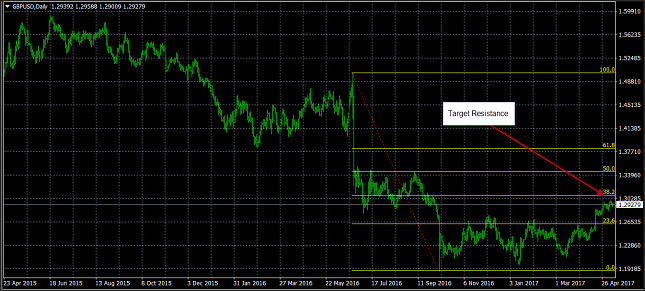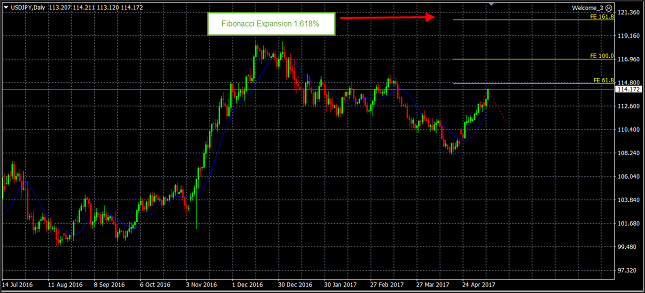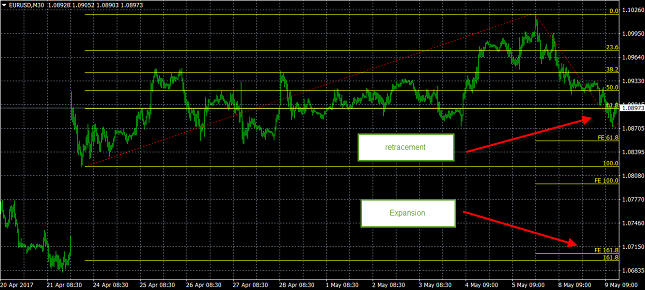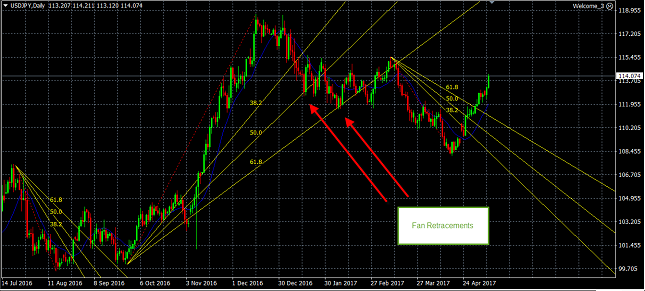FXEMPIRE
AllCorona Virus
Stay Safe, FollowGuidance
World
99,710,759Confirmed
2,137,559Deaths
71,683,987RecoveredFetching Location Data…As currency pairs fluctuate in the ever changing forex markets, it’s important to be able to forecast support and resistance levels, and where an exchange rate might reverse. Determining where to take profit on a position or stop loss on a trade, takes time to master. It’s important to fill your trading arsenal with as many tools as possible to help you foreshadow changes before they occur, or at least have an idea where the market is headed. While you might have found a system or technique that has help you perform well over time, studying the habits of price action will allow you to forecast what the market might do in the future. One of the most prolific techniques you can use to define future targets is a Fibonacci sequence.

## What is the Fibonacci Numbers and the Golden Ratio?

Leonardo Pisano Bogolla is the founding father of the Fibonacci sequence. He was an Italian mathematician from the city of Pisa, and is credited with introducing the Fibonacci sequence. The sequence is derived from a mathematical view of nature and begins with zero, and then 1, and generates a new number to the sequence by adding the sum of the two prior numbers. After 1, the next number is 2, and then 3, and 5, and so on adding the prior two numbers together to generate the next number in the sequence. The Fibonacci sequence is never ending and has several unique properties.

One of the most important of these unique properties is the ratios that are generated from the numbers within the sequence. These ratios, referred to as golden ratios, are created in 2-distinct manners. If you divide a number by the previous number within the sequence, the ratio that is created is 1.618.  For example, if you divide 13 by 8, the ratio is 1.618, and if you divide 21 by 13, you get the same ratio. A second important ratio occurs if you divide a number by the number 2-places higher.  Here you will get a ratio of 0.382.  For example, if you divide 3 by 8, you get 0.382.

The Fibonacci ratios are used to find target levels by measuring the distance that a security has traveled and attempting to determine the reversal that will occur using the ratio’s as a predictor of how far the market will rebound.

## Fibonacci Retracement Levels

Fibonacci retracement levels are constructed by using the golden ratios, and describe a potential target retracement level, after a certain security has increased or decreased. The two most commonly used ratios are the 38.2% ratio, which is derived from dividing the current number in the Fibonacci sequence by the number two places higher, or the 61.8% retracement level which is calculated from dividing the current sequence number by the prior number in the sequence.

A Fibonacci retracement level can be used as support or resistance levels. You can evaluate the region that is targeted by the retracement as a place to enter a counter trade of the current trend, or a place to take profit. If the price of an exchange rate continues to slice through a Fibonacci level, you can view the move as a continuation of a trend. Once a pullback starts you can find specific Fibonacci levels to target the new advance.An example of a retracement is seen on the chart of the GBP/USD currency pair.  Here prices dropped in May of 2016 following the UK referendum on Brexit hitting a low in September 2016. Once the low was in place, you could use a Fibonacci retracement tool, to target the 38.2% retracement level which is seen near 1.3050.  If the exchange rate moves through this level, the next likely target is the August highs which coincide with the 50% retracement level. While the 50% level is not a Fibonacci retracement level, and is taken from Dow Theory, it is often incorporated into Fibonacci tools.

## Fibonacci Extensions

A Fibonacci extension uses the concept of extrapolation to determine future target levels based on historical movements in prices. The process uses the same Fibonacci sequence that is used to create the retracements, but instead of providing levels where a security will retrace to in the future, following a move higher or lower, the extension will provide targets for the continuation of a move.For example, a Fibonacci extension was added to the chart of the USD/JPY extrapolating from the lows near 108.25 to the highs near 114.20.  This generated a Fibonacci extension by multiplying the change in the currency pair by 1.618%, creating a target near 1.21. You can use the extension to generate take profit levels after an exchange rate breaks out, or as a place to stop loss on a short of the currency pair.

## How to Trade the Fibonacci Retracement and Extension?

You can use a Fibonacci retracement on all time frames, from monthly to intra-day. Since markets consolidating more than they trend, this type of analysis can be very helpful to a day trader who is looking to define specific ranges to trade around.

For example, the 30 minute chart of the EUR/USD shows a gap in late April and a slow climb to the highs in early May. The retracement from the gap low to the May highs shows a retracement back to the levels where the EUR/USD is consolidating. The lows, which are support are just below near 1.0870, which was targeted twice.  his might be strong support, as the Fibonacci retracement shows that the move has retraced 61.8%.

The chart also shows where the exchange rate could target as support, if the currency pair breaks through support levels. The Fibonacci extension shows that a 1.618% extension level would take priced down to the 1.0715 region. If you are relying on a trading strategy that uses support and resistance levels, and ranges, then incorporating Fibonacci retracements and extensions can help you to tune your targets.Fibonacci Arcs

Fibonacci Arcs are semi-circles that are constructed from the Fibonacci sequence combining the form of an arc into the process of defining support and resistance levels. The arcs are generated by extending a point from a line that forms the basis between two or more points. There are multiple arcs in a Fibonacci arc drawing but all are based of the golden Fibonacci ratios of 38.2% and 61.8%. There is however a second or middle arc is set at 50%. This arc is formulated from Dow Theory.

Similar to the retracement, the arc offers a way to describe support and resistance levels. After an advance or decline of a currency pair, the Fibonacci arcs are created by measuring the base line that extends from trough to peak.  The radius of the arc are measured using the 38.2%, 50%, and 61.8% Fibonacci levels. The arc that is create marks support and resistance levels, where price might correct after an advance or decline in the exchange rate. After a drop in the price of a security, the Fibonacci arc is used to estimate resistance for a counter trend move.

Similar to evaluating a Fibonacci retracement, the first step in evaluating a Fibonacci arc is to pick the peak and trough of move of an asset. You would then draw the line from trough to peak for an advance and the reverse for a decline.

The Fibonacci Arcs differ from retracements and expansion because they incorporate time into their equation. Retracements and extensions are purely based on price points while the arc has an angle from the base Line after an advance extends from trough to peak at an angle that is dependent on elapsed time. The slope and length of the line depend on changes in both price and time.

Similar to retracements, arcs are used as retracement tools as well as target tools that can be used to find a take profit level. This means it can be used as a risk management devise to find support and resistance. If the market retraces after an advance it is deemed a correction that will eventually find support above the beginning of the advance.

## Fibonacci Fan Lines

Fibonacci Fan lines are trend lines are also generated from using the Fibonacci sequence, and are based on the gold ratio as well as the 50% level from Dow Theory.  A fan line will extend down if you are measuring a downtrend, and extend outward producing resistance levels which you can use to determine the potential rebound.  Fan lines also incorporate time into their equation and will show you potential retracement levels and when they should occur.The daily chart of the USD/JPY shows different retracement levels that are a function of time. The large move up in the currency pair from September 2016 to December 2016 generated a fan retracement that showed 2 specific periods where the exchange rate bounced, hitting support before moving lower. You could have used these as take profit levels, or even levels to purchase the currency pair looking for a bounce in the exchange rate. These fan lines can then be used to estimate support and resistance areas as well as target zone where the market might reverse.

It’s helpful to use a fan, which is a style of retracement once a pullback starts, so you can target levels of support or resistance. Fans can be used on any time frame and are helpful for intra-day forex traders as it will help you target levels in the future that are take profit or stop loss levels.

Fibonacci Fans, similar to retracements and arcs are used to identify potential reversal points. These metrics assume the move is corrective in nature and that the market will bounce and target these levels. Fibonacci Fan lines allow users to anticipate the ending points for these counter-trend moves.

## How to use Fibonacci in Trading?

Fibonacci retracements, extensions, arcs and fans are tools that can be used to help generate support and resistance levels. These tools should be used in conjunction with other trading tools whether they are your fundamental view or another technical analysis indicator such as momentum or trend following studies. You can incorporate Fibonacci tools into your trading strategy to find levels to enter a trade, as well as exit a trade based on projected targets that are forecasted by the Fibonacci mechanism. Fibonacci can also be integrated with other technical analysis tools such as MACD or elliot waves.

## Summary

Fibonacci retracements, arcs and fans are excellent tools you can use to help pin point an entry point into a trade along designating stop loss and take profit levels. By using this mathematical formula, you can combine this relationship with other technical analysis tools to help yourself find the best levels of support and resistance. By combining Fibonacci retracements, extensions, arc and fans with other technical analysis indicators, you can enhance your trading strategy, by forecasting levels where a currency pair is poised to reverse.

## Tips From the Expert

• Use Fibonacci retracements to augment your ability to find support and resistance levels
• You can use retracements to pinpoint take profit and stop loss levels
• Even if you don’t use these retracements, understanding what users might be doing is very helpful
• Fibonacci extensions are a helpful way to target how far a security will travel
• You can incorporate time into the equation by using Fibonacci fans
Don't miss a thing!

## Latest Articles

See All

See All